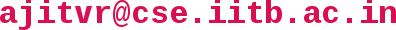## CS663 - Digital Image Processing

Office: SIA-218, KReSIT Building
Email:Lecture Venue: LC 101
Lecture Timings: Slot 10, Tuesday and Friday 2:00 to 3:30 pm
Instructor Office Hours (in room SIA-218): Tuesday and Friday 5:00 to 6:00 pm, or by appointment
Teaching Assistants: B S Deepak, Hc Lalduhbera, Anshuman Dhuliya, Thyagarajan Radhakrishnan

## Lecture Schedule:

(Continuing from the previous set of lectures taken by Prof. Suyash Awate)

### Content of the Lecture

16/09 (Tue)
• Face recognition: intro; Principal components analysis for face recognition (eigenfaces): intro, concept of covariance matrix, description of algorithm and its computational complexity
19/09 (Fri)
• Faster version of eigenfaces algorithm; meaning of principal components analysis (PCA); derivation of PCA and hence eigenfaces; selection of 'k' in eigenfaces algorithm
23/09 (Tue)
• Person-specific eigenspaces; explanation of Lagrange multipliers; PCA for compression; Introduction to the Singular value decomposition; applications in image compression; Eckart Young theorem introduction
26/09 (Fri)
• SVD, SVD and its relationship to eigenvectors and eigenvalues, SVD-based image compression, Eckart Young theorem, geometric interpretation; Introduction to image restoration
30/09 (Tue)
• Image restoration: defocus blur, motion blur model; inverse filter and its problems; spread spectrum blurs: cameras with coded apertures and flutter-shutter camera
7/10 (Tue)
• Wiener filter: aim, assumptions, derivation, interactive Wiener filter; Concept of convolution as multiplication with a circulant matrix; Concept of computing inverse Fourier transform as multiplication with Fourier matrix; deconvolution by matrix inverse and its relation to Fourier-based inverse filter; block-circulant matrix; Fourier matrix for 2D signal as Kronecker product of two Fourier matrices for 1D signals
10/10 (Fri)
• Use of PCA for image denoising: ideas using non-local means (patch-based filtering), PCA and Wiener filter; Regularized least squares deblurring using image Laplacian as a regularizer
14/10 (Tue)
• Image segmentation: problem introduction, K-means clustering, concept of medoid; Histograms and Kernel density estimation; cluster centers as modes of a probability density function
17/10 (Fri)
• Histograms and Kernel density estimation; cluster centers as modes of a probability density function; gradient ascent; mean shift algorithm; mean shift as adaptive gradient ascent; mean shift for image smoothing and its relationship to bilateral filtering; mean shift for image segmentation (and its caveats); selection of smoothing parameters in kernel density estimation.
21/10 (Tue)
• Edge detection: types of edges (ramp, step, roof); digital derivative operators in 1D and 2D, zero crossing of second derivative, image Laplacian and its rotational invariance; Robert and Sobel operators; Marr-Hildreth edge detector; Canny edge detector: gradient magnitude and orientation computation, non-maximal suppresion and hysterisis thresholding.
Hough transform for detection of lines, circles and ellipses
28/10 (Tue)
• Image compression paradigms: lossy and lossless, motivation for lossy compression; Overview of JPEG standard: discrete cosine transform, its relationship to discrete Fourier transform, relationship between discrete cosine transform and PCA.
31/10 (Fri)
• Relationship between DCT and DFT; steps of JPEG: quantization, Huffman encoding and run length encoding; JPEG for color images: YCbCr color space, and PCA on RGB values; Modes of JPEG compression